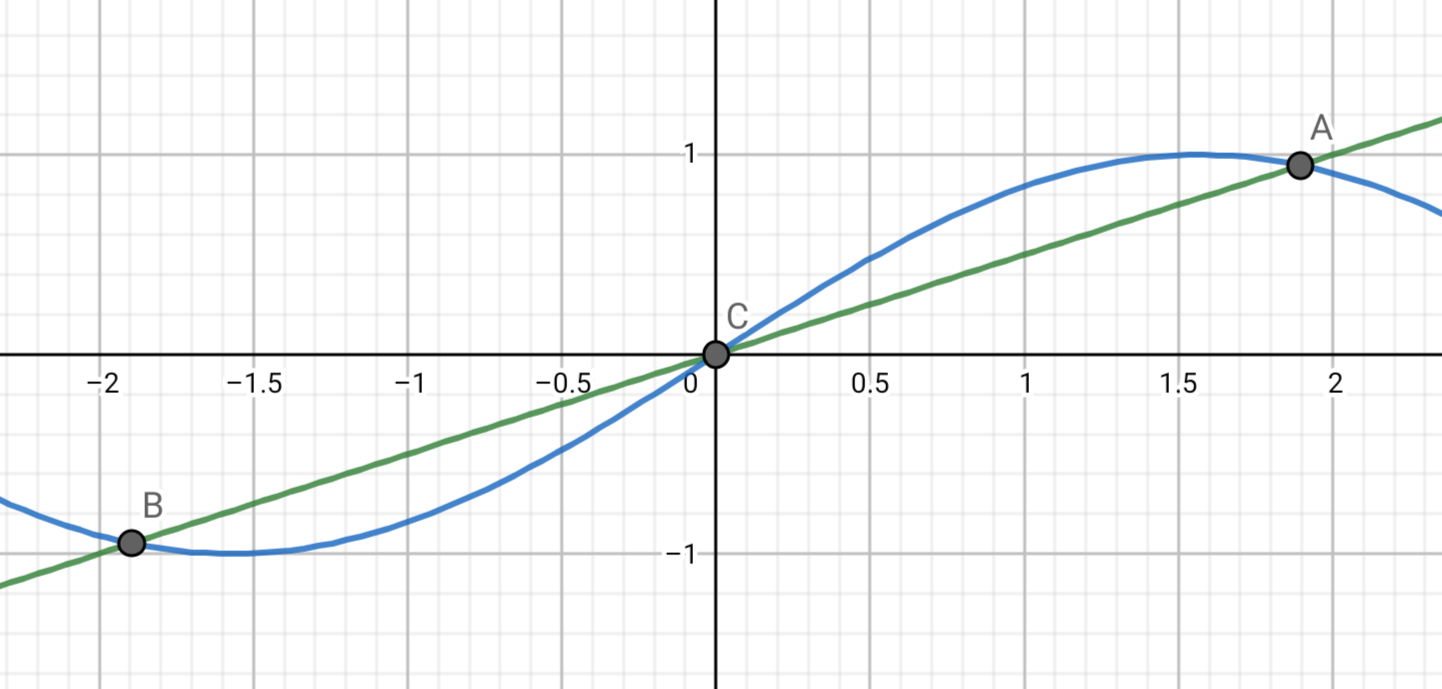### Line of Thought Tangent to Work

I have a lot of downtime at school, so I've been revisiting one of my favorite high-school subjects: calculus.

My study is decidedly nonsystemic. I've been doing problem sets from a Korean calc textbook that my host sister gave me, but I spend the bulk of my practice sessions just making up problems for myself. I try to come up with things I know I can't do, then look up the solution (everything's online) and puzzle through it step by step. I suppose that's because beyond learning calculus, I have a secret goal in mind: to think outside the box about pedagogy.

A math puzzle I created this week reminded me that a small change can turn something straightforward into a maze of algebra. Consider a function like y = sin(x). If I want to find the intersection between this function and a horizontal line like y = 3/4, it's trivial: I take arcsin(3/4). But what about the intersection between y = sin(x) and a sloped line, like y = x/2? A look at the graph shows there are clearly three points of intersection: one at x = 0, and then two others near x = ±1.9.But you can kick the variables around all day and never get an answer in closed form. The exact solution to this problem is x such that x/2 = sin(x). WolframAlpha computes an approximate answer using (probably) Newton's method.

It's not hard to imagine a careless math teacher including a problem like this on an exam, thinking they'd make an answer key later. After all, finding the intersection of two curves is a standard precalc problem. I can even make it look like a geometry problem: Find θ such that A1 = A2. Figure not to scale.Clearly, there's only one angle you could put there (besides 0) that would produce such a shape. That just a bit of algebra and trigonometry, right? But the trig reduces to θ/2 = sin(θ). If a high-school geometry teacher gave this problem to students, the mediocre ones would be baffled and the motivated ones would be crushed.

To put it in general terms, in pedagogy, there's a fine line between a healthy challenge and a self-esteem killer. In my English class, I often include words on my handouts that I'm certain my students won't know. It's not because I want to remind them that they don't know the meaning of underestimate. It's because I want them to practice overcoming the sense of panic that arises when you see a long, confusing word at some crucial position in the sentence. Once they sit in the feeling of panic for a moment, the students' brains start working. They try breaking the word up into meaningful parts, guessing the meaning from context, or asking a friend what they think—all valid techniques that real English speakers use when they encounter a new word in the wild. This is possible because I chose underestimate, a very guessable word. I would expect a much lower rate of success with, say, ombudsman. In mathspeak, it's a challenge problem, but it's one I know my students can do. It's the arcsine problem, not the transcendental equation.